# Implementation of a Smart Antenna Base Station for Mobile WiMAX Based on OFDMA

## Abstract

We present an implementation of a mobile-WiMAX (m-WiMAX) base station (BS) that supports smart antenna (SA) functionality. To implement the m-WiMAX SA BS, we must address a number of key issues in baseband signal processing related to symbol-timing acquisition, the beamforming scheme, and accurate calibration. We propose appropriate solutions and implement an m-WiMAX SA BS accordingly. Experimental tests were performed to verify the validity of the solutions. Results showed a 3.5-time (5.5 dB) link-budget enhancement on the uplink compared to a single antenna system. In addition, the experimental results were consistent with the results of the computer simulation.

## 1. Introduction

Modern mobile communication requires not only a high data rate transmission but also a relatively fast mobility. The mobile WiMAX (m-WiMAX) based on orthogonal frequency division multiple access (OFDMA) is believed to be a solution that addresses both of these requirements . Moreover, the application of smart antenna (SA) technologies to OFDMA is regarded as a key solution for increasing the data rates and the mobility of fourth generation (4G) wireless communication systems operating in frequency-selective fading environments. However, there are several things to consider in baseband signal processing when implementing SA systems in OFDMA. These include the performance of symbol-timing acquisition, the beamforming scheme, and accurate calibration.

The SA system enlarges cell coverage through beamforming. However, to obtain effectively enlarged cell coverage, performance of the initial acquisition and symbol synchronization should also be enhanced. Since initial acquisition is performed prior to calculating the weight vector, an algorithm to enlarge the acquisition coverage is required. Moreover, in the contention-based ranging used in m-WiMAX, since classification of the ranging signal by the user is impossible prior to decoding, it is difficult to properly apply a weight to the desired ranging signal.

Various beamforming algorithms for OFDMA communications have been investigated [2, 3]. However, most of the research focuses on beamforming per subcarrier using the conventional single-carrier beamforming algorithm. This approach causes high computational loads and increases system complexity.

The calibration technique is essential for the SA system to apply a proper beamforming weight to the transmission. Without an accurate calibration technique, the advantages of SA technology cannot be provided in the downlink . More specifically, even if the optimal weight vector is computed from the received signal, downlink (DL) beamforming can never be optimized without accurate calibration. The primary reason is that the beamforming parameter for the DL is, in most cases, heavily dependent upon the parameter values computed during the uplink (UL). Thus, the overall communication quality of the SA base-station (BS) system cannot be improved without a proper calibration technique.

In this paper, we propose solutions for these problems and implement an m-WiMAX SA BS accordingly. In Section 2, we propose our solutions, and Section 3 shows the implementation of the m-WiMAX SA BS. Each signal-processing module is described in detail in this section. The performance of the m-WiMAX SA BS is presented in comparison to the conventional single-antenna BS in Section 4, and computer-simulation results are shown to verify our experimental results. Finally, we conclude this paper in Section 5.

## 2. Considerations for Implementation of the m-WiMAX SA BS

This section addresses some essential problems that must be considered when implementing the m-WiMAX SA BS. These include the performance of symbol-timing acquisition, an optimized beamforming scheme, and accurate calibration. For SA BS to provide effective coverage, the coverage of the symbol-timing acquisition must be enhanced. The optimized beamforming scheme is essential to implement an SA BS. Finally, to provide proper downlink and uplink beamformings, a pragmatic procedure for automatic calibration is required for the SA BS. In the following subsections, we propose solutions to these problems.

### 2.1. Ranging Processing

The problem of ranging arises because the propagation delays between the SA BS and each of the mobile stations (MSs) in a given cell is different, so the arrival time of the signal associated with each of the subscribers cannot be the same. Beamforming gain can be obtained in the SA BS only when symbol time synchronization is performed properly. Thus, proper symbol time synchronization is a prerequisite if the SA BS is to enhance communication capacity and cell coverage.

Time synchronization, which is used to compensate for differences in propagation delays, is referred to as "ranging" in the mobile-WiMAX system. Each subscriber randomly selects a ranging code, allocates that code to the ranging channel, and transmits it in the form of a ranging symbol. The BS then checks whether or not the ranging code has been transmitted in a given uplink frame at each frame time throughout the code detection procedure. When the BS detects the ranging code transmitted by a subscriber, it finds the ranging code index and estimates the propagation delay associated with that MS.

Figure 1 illustrates the ranging channel receiver in an m-WiMAX SA BS. This algorithm is less complex and more efficient than conventional correlation-based algorithms [5, 6]. In other words, for an-subcarrier m-WiMAX system, the conventional correlation-based algorithm requirescomplex multipliers while the proposed ranging algorithm requires only. Assuming that the propagation delay of the ranging symbol arriving at the BS is, the receiving (RX) signal of each antenna is not retrieved correctly because of the propagation delay. Based on the correlation characteristics of the pseudorandom binary sequence (PRBS) and the circular shift property of the discrete Fourier transform operator, after the fast Fourier transform (FFT) operation, the signal of each antenna is descrambled using the ranging code transmitted by the target subscriber and then becomes a rectangular function with its phase rotated in proportion to the propagation delay. After taking the inverse-FFT (IFFT) of the descrambled signal, the absolute value of the signal of each antenna is summed. This value is denoted asand has its maximum value when. The structure of the ranging channel receiver shown in Figure 1 provides a diversity gain in both ranging code detection and propagation delay estimation because the detection variable is obtained through a noncoherent combination at each antenna path.

The signal received through antenna path,, can be written as(1)

whereis the time-domain symbol obtained as the result of an IFFT at subscriber m,is the distance between theth and reference antenna element,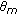is the direction of arrival (DOA), andis the wavelength of the received signal at its carrier frequency. For simplicity, but without loss of generality, we have assumed that there are no other user signals. The FFT of (1) can then be written as(2)

whereis length of the ranging code. To apply the proposed algorithm, the received signal shown in (2) is descrambled with the ranging code,, and processed with the IFFT operator as shown in Figure 1. In the case of, the result of the IFFT operation can be written as(3)

The received signal shown in (3) is a complex Gaussian random process with a mean of, which implies that the detection variable obtained at each antenna channel,, is a noncentral chi-square random process with two degrees of freedom. The detection variable of the array antenna system consisting ofantenna elements is consequently a noncentral, chi-square distributed random variable with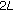degrees of freedom, and can be written as(4)

where,is the modified Bessel function of the first kind of order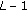, and where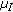and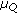denote the real and imaginary parts of. The mean and variance of the detection variable in an array system consisting ofantenna elements are expressed as(5)

wheredenotes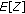. The mean and variance of the detection variable increase linearly in accordance with the number of antenna elements, as shown in (5). This means that the SNR of the ranging code detector increases in proportion to, where the SNR of the ranging channel receiver is defined as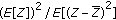.

On the contrary, if the signal of each antenna is descrambled with a code that is different from the one transmitted by the target subscriber,approaches zero due to the correlation characteristics of the ranging codes.

Figure 2 illustrates the probability,, of estimating the exact propagation delay provided by the proposed ranging channel receiver in terms of the number of antenna elements. As shown in the figure, the performance of the propagation delay estimation improves as the number of antenna elements increases. For a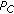of at least 99%, the minimumof the communication channel with an array system of four antenna elements is about −4.4 dB. Compared to the BS consisting of a single-antenna element, the BS consisting of four antenna elements provides a performance enhancement of approximately 6.0 dB in the SNR.

### 2.2. Beamforming Scheme

The conventional beamforming algorithms for OFDMA use samples in time to estimate the statistical characteristic of the spatial channel [2, 3]. This approach avoids the effect of frequency selective fading. However, it is difficult to obtain enough samples to estimate the statistical characteristic of a spatial channel in an m-WiMAX waveform which is a packet-based communication. Note that the spatial-channel basis is independent of both time and frequency in narrowband communications. Therefore, we can obtain enough samples to estimate the spatial-channel basis in both the time and frequency domains.

In this paper, we propose a beamforming scheme that uses samples from both the time and frequency domains to estimate a spatial-channel basis which is used as the beamforming-weight vector. The processing procedure for the proposed scheme is depicted in Figure 3. In Figure 3, n and k are the time and frequency indices, and N and K are the total number of pilot subcarriers in the time and frequency domains of a given packet. Compared to conventional beamforming, the biggest advantage of the proposed scheme is that more samples can be obtained from the given OFDMA symbols (i.e.,) to calculate the weight vector. The second advantage is that the delay for converging the weight vector calculated by the adaptive algorithm is reduced. In this paper, the Lagrange multiplier-based algorithm  is used for the beamforming scheme.

Figure 4 shows the performance comparison between the conventional beamforming and the proposed beamforming when the m-WiMAX packet consists of 15 OFDMA symbols. In this computer simulation, quadrature phase-shift keying (QPSK) was used as the modulation and the SA BS had a four-element array. The channel environment for the simulation was a Rayleigh fading channel of which the maximum Doppler-frequency component was 266.77 Hz. Note that the channel environment did not correspond to the experimental test in Section 4. As shown in Figure 4, the performance of the conventional beamforming was reduced by 1.2 dB in bit error rate (BER) due to the lack of samples.

### 2.3. Calibration

The problem of calibration occurs because the phase characteristics of the radio frequency (RF)/intermediate frequency (IF) chains associated with each antenna are different in both the receiving (RX) and transmitting (TX) modes. Several calibration techniques for the SA system have been proposed . Of these techniques, we chose to use  because it offers simple and accurate calibration. Although the experimental data in  was obtained using the CDMA2000 1x standard, it is noteworthy that this technique can be applied to the OFDMA standard. Another advantage is that this technique can be applied while the SA system is operating.

The chosen calibration technique requires the installation of an additional antenna which is used to TX or RX a test signal to or from each antenna element for RX and TX calibrations. This additional antenna transmits the test signal through an RX carrier frequency and receives the test signal through a TX carrier frequency. The calibration is performed separately, since the RX and TX modes exist separately in the frame format of mobile WiMAX. By using a test signal orthogonal to the RX/TX signal, the influence on the SA BS can be minimized when the calibration operation is performed.

The RX path calibration was performed using the following procedure.

1. (1)

The additional calibration antenna generates and transmits a test signal.

2. (2)

Each RX path in the SA system receives the signal simultaneously.

3. (3)

The calibration processor calculates a calibration value for each RX path in the SA system.

An exact numerical analysis of the procedure is given in . The phase delay of the wireless path between each antenna and the additional antenna can be calculated by making a connection between each antenna path and the additional antenna path with a cable. The phase difference between each antenna RX path is obtained by correlating the received signal from each antenna path with the test signal.

The TX path calibration is performed separately from the RX path calibration using the following procedure.

1. (1)

The calibration processor generates N (the number of antenna elements) orthogonal test signals for each TX path of the SA system.

2. (2)

Each path transmits the signals.

3. (3)

4. (4)

The calibration processor calculates the calibration value for each TX path of the SA system.

As shown in , the phase difference between each antenna and the reference antenna is almost eliminated using the calibration. As a result, a proper beam pattern can be obtained.

## 3. Implementation of the m-WiMAX SA BS

Figure 5 shows the baseband-SA modem for the m-WiMAX SA BS. The SA modem consists of eight fixed point digital signal processors (DSPs), two field programmable gate arrays (FPGAs), and a general purpose processor (GPP). In the modem, three DSPs exist for redundancy and are not used for signal processing. Five DSPs are used for encoding/decoding, beamforming, calibration, and ranging processing. Two FPGAs perform FFT/IFFT and permutations. Finally, the GPP is used for medium access control (MAC) to interface between the SA BS and the network. The detailed functionality of each device is described as follows.

Figure 6 shows the signal flow of the baseband as well as the allocation of the signal processing components to the devices in the SA modem. In the case of UL, the received signal is fed into the frontFPGA via low-voltage differential signaling (LVDS). The frontFPGA removes the CP of the received OFDMA symbols and passes it to the rearFPGA. The rearFPGA performs FFT, tile permutation, and UL weighting. The ranging code is also descrambled in the rearFPGA. The descrambled ranging channel is passed to RNG_DSP for estimating the symbol timing, and the data channel is passed to UL_DSP for decoding. The beamforming-weight vector is calculated by BF_DSP using the pilots embedded in the permutated data channel. The BF_DSP returns the weight vector to the rearFPGA. The weight vector is used for both UL and DL, since the m-WiMAX is operated in time-division duplex (TDD) mode. The decoded data is analyzed in MAC_GPP and sent to the network. In DL, the MAC protocol data unit (PDU) is fed into DL_DSP for encoding. The encoded data is passed to the rearFPGA for DL weighting, cluster permutation, and IFFT. The frontFPGA receives the OFDMA symbol and adds the CP. When the DL frame clock is enabled, the frontFPGA sends the OFDMA symbol to the intermediate frequency (IF) module via LVDS. The calibration is performed independently of UL/DL processing. The result of the calibration is multiplied with the weight vector in BF_DSP to compensate for the amplitude and phase differences among the RF/IF chains.

Figure 7 describes how the signal processing is performed in synchronization with the system clock. The system clock (sysClk in Figure 7) generates a 10 MHz pulse. The frmSync is raised at the beginning of every frame duration, and UL_DL is toggled at every DL and UL duration. In Figure 7, we can see that all signal processes in Figure 6 are performed in parallel.

Figure 8 is a photograph of the up-down converter unit (UDCU) employed in our SA BS. The UDCU consists of an analog-to-digital (A/D) converter, a digital-to-analog (D/A) converter, an Up/Down converter, and automatic gain control (AGC). When transmitting, the digital data from the SA modem is converted to the corresponding analog signal through D/A conversion. This analog signal is converted to an RF signal via the Up-converter. Then, the RF signal is transmitted through the front-end unit (FEU). When receiving, the received signal obtained from the FEU is first fed into the AGC. Then, the output of the AGC is converted to a digital signal which is sent to the SA modem.

The FEU, shown in Figure 9, includes a TDD switch and a low-noise amplifier (LNA). The TDD switch isolates the transmit and receive signals from each other in accordance with the DL and UL duration. The LNA amplifies the received signal with a noise level that is as low as possible.

The array antenna was implemented using five patch-type elements. The element spacing was a half-wavelength (6.52 cm). Four elements were used for transmitting and receiving signals, and the other element was used for calibration.

The signal processing modules presented in this section were integrated into the m-WiMAX SA BS. A photograph of the entire SA BS is provided with a description of the experimental environment in the next section.

## 4. Experimental Results

In this section, experimental results obtained from the implemented m-WiMAX SA BS are presented, including the symbol-timing estimation probability for the ranging process, the accuracy of the phase-delay compensation for the calibration, and throughput. In addition, various computer simulations supported the validity of our experimental results.

Figure 10 shows the experimental environment that included the implemented m-WiMAX SA BS, a six-element array antenna, mobile-station emulator, signal generator, spectrum analyzer, and server and client laptops which were connected to the BS and MS via Ethernet. Four elements of the array antenna were used to transmit and receive the m-WiMAX signal, and the other element was used for the proposed calibration. An additional element, connected by the spectrum analyzer, was used for measuring the signal-to-noise ratio (SNR) at the RF input of the SA BS. The signal generator radiated additive white Gaussian noise for handling the SNR. To compare the performance between the SA BS and the conventional single antenna BS, two SA modems for the SA BS were used simultaneously. One SA modem was set to the conventional single-antenna mode by receiving the signal from an element of the array antenna, and the other modem was set to the SA mode. The system parameters used in this test are summarized in Table 1.

Figure 11 shows a comparison of the symbol-timing estimation probability of the conventional ranging process and the proposed ranging process. The experimental results were obtained by averaging the measurements during 10 000 frames, that is, a 50-second period. In addition, the experimental results coincided well with the results of computer simulations which were calculated by compensating for the SNR in Figure 2. As shown in Figure 11, the proposed ranging process provided about a 5.7 dB enhancement in symbol-timing estimation probability compared to the conventional ranging process.

Figures 12 and 13 show the measurements of the relative phase differences between each RF/IF chain and a reference RF/IF chain before and after the proposed calibration. As shown in Figure 12, the relative phase delay at each RF/IF chain differed from the others but remained nearly constant over time. From the measurements, we observed that the phase delay of the RF/IF chain associated with each antenna element remained steady for a duration of over 500 symbols. Figure 13 shows the phase delay after the proposed calibration. The standard deviation of the residual phase error of the relative phase delay at each antenna element was 2-3° and remained steady for five hours. Figure 13 shows that the proposed calibration technique eliminated the phase difference of the RF/IF chain associated with the antenna elements.

Finally, Figure 14 shows the measured uplink throughput of the conventional single-antenna BS and SA BS. The experimental results were averaged over five minutes per given SNR. To measure the throughput of both BSs, a movie file was uploaded from the client laptop, which was connected to the MS, to the server laptop connected to the BS. In other words, the experiment was performed with packet-based communication. As shown in Figure 14, the proposed beamforming scheme provides a 5.5 dB link-budget enhancement. These results mean that the proposed beamforming scheme can be implemented. In addition, the experimental results are consistent with the results from the computer simulation.

## 5. Conclusion

In this paper, we addressed three key issues in implementing the m-WiMAX SA BS: ranging, beamforming, and calibration.

First, the proposed ranging process significantly reduced calculation loads using IFFT instead of a correlation operation. Moreover, the proposed process achieved diversity gain in the received signals from each antenna path.

Second, the proposed beamforming scheme addressed the lack of samples in OFDM-based packet communications. The proposed scheme used time and frequency samples for obtaining the statistical properties of the spatial channel.

Finally, the calibration method, which can be applied while the SA system is operating, was proposed. Although additional antenna chains are required, the proposed method provided fast and accurate performance.

The experimental results and computer simulations verified the validity of these solutions. As shown in Section 4, the proposed solutions can be applied to the m-WiMAX SA BS. In addition, the m-WiMAX SA BS increased the link-budget by 5.5 dB.

It should be noted that the experiments described in this paper represent lab tests only, which might be quite different from the outdoor environments in which m-WiMAX is used. As shown in Figure 10, the MS in our lab tests was located just 4-5 meters away from the BS in a direct line of sight. Since a mobile fading environment cannot easily be set up in the laboratory, we checked the proposed beamforming scheme in fading environments through various computer simulations. As shown in Figures 2 and 4, it is clear that the proposed beamforming scheme provided a remarkable improvement in mobile fading environments as well as in the static circumstances of the lab tests. Another limitation of the experimental tests was that the calibration performance was not verified in the throughput tests shown in Figure 14. Note that as the calibration was used for downlink beamforming, the uplink performance shown in this paper does not confirm the validity of the proposed calibration procedure except that the phase differences at each antenna channel were equalized as shown in Figures 12 and 13. Future tests could include the downlink measurements to verify the actual performance of the proposed calibration procedure.

## References

1. WiMAX Forum : Mobile WiMAX—part I: a technical overview and performance evaluation. http://www.wimaxforum.org/

2. Li Y, Sollenberger NR: Adaptive antenna arrays for OFDM systems with cochannel interference. IEEE Transactions on Communications 1999, 47(2):217-229. 10.1109/26.752127

3. Chen Y-F, Li C-P: Adaptive beamforming schemes for interference cancellation in OFDM communication systems. Proceedings of the 59th IEEE Vehicular Technology Conference (VTC '04), May 2004, Milan, Italy 1: 103-107.

4. Wennström M, Öberg T, Rydberg A: Effects of finite weight resolution and calibration errors on the performance of adaptive array antennas. IEEE Transactions on Aerospace and Electronic Systems 2001, 37(2):549-562. 10.1109/7.937468

5. van de Beek JJ, Sandell M, Börjesson PO: ML estimation of timing and frequency offset in OFDM systems. IEEE Transactions on Signal Processing 1997, 45(7):1800-1805. 10.1109/78.599949

6. Fu X, Minn H: Initial uplink synchronization and power control (ranging process) for OFDMA systems. In Proceedings of the IEEE Global Telecommunications Conference (GLOBECOM '04), November-December 2004, Dallas, Tex, USA. Volume 6. IEEE Communications Society; 3999-4003.

7. Choi S, Shim D: A novel adaptive beamforming algorithm for a smart antenna system in a cdma mobile communication environment. IEEE Transactions on Vehicular Technology 2000, 49(5):1793-1806. 10.1109/25.892584

8. Litva J, Lo TK: Digital Beamforming in Wireless Communications. Artech House, Norwood, Mass, USA; 1996.

9. Mano S, Katagi T: A method for measuring amplitude and phase of each radiating element of a phased array antenna. Journal of the Institute of Electronics and Communication Engineers of Japan 1982, 65(5):555-560.

10. Nishimori K, Cho K, Takatori Y, Hori T: Automatic calibration method of adaptive array for FDD systems. Proceedings of the IEEE Antennas and Propagation Society International Symposium (APS '00), July 2000, Salt Lake, Utah, USA 2: 910-913.

11. Hyeon S, Yun Y, Choi S: Novel automatic calibration technique for smart antenna systems. Digital Signal Processing 2009, 19(1):14-21. 10.1016/j.dsp.2007.10.006

## acknowledgments

This work was partly supported by the IT R&D program of MIC/IITA (2007-S001-01, Implementation of Advanced-MIMO system) and the HY-SDR research center at Hanyang University, Seoul, South Korea under the ITRC program of MIC, South Korea.

## Author information

Authors

### Corresponding author

Correspondence to Seungwon Choi.

## Rights and permissions

Reprints and Permissions

Hyeon, S., Lee, C., Shin, Ce. et al. Implementation of a Smart Antenna Base Station for Mobile WiMAX Based on OFDMA. J Wireless Com Network 2009, 950674 (2009). https://doi.org/10.1155/2009/950674

• Revised:

• Accepted:

• Published:

• DOI: https://doi.org/10.1155/2009/950674

### Keywords

• Medium Access Control
• Orthogonal Frequency Division Multiple Access
• Antenna Element
• Automatic Gain Control
• Smart Antenna